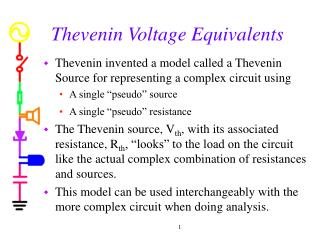# Thevenin Voltage Equivalents - PowerPoint PPT PresentationDownload PresentationThevenin Voltage Equivalents

Thevenin Voltage EquivalentsDownload Presentation## Thevenin Voltage Equivalents

- - - - - - - - - - - - - - - - - - - - - - - - - - - E N D - - - - - - - - - - - - - - - - - - - - - - - - - - -
##### Presentation Transcript

1. Thevenin Voltage Equivalents • Thevenin invented a model called a Thevenin Source for representing a complex circuit using • A single “pseudo” source • A single “pseudo” resistance • The Thevenin source, Vth, with its associated resistance, Rth, “looks” to the load on the circuit like the actual complex combination of resistances and sources. • This model can be used interchangeably with the more complex circuit when doing analysis. 1

2. The Function Generator Model • Recall that the function generator has an internal impedance of 50 Ohms. • Could the internal circuitry of the function generator contain only a single source and one resistor? • This is actually the Thevenin equivalent model for the circuit inside the function generator 2

3. Thevenin Model Load Resistor 3

4. Note: • We might also see a circuit with no load resistor, like this voltage divider. 4

5. Thevenin Method A • Find Vth (open circuit voltage) • Remove load if there is one so that load is open • Find voltage across the open load • Find Rth (Thevenin resistance) • Set voltage sources to zero (current sources to open) – in effect, shut off the sources • Find equivalent resistance from A to B B 5

6. Find Vth • Remove Load A B A B 6

7. Find Vth • Let Vo=12, R1=2k, R2=4k, R3=3k, R4=1k 7

8. Find Rth • Short out the voltage source (turn it off) & redraw the circuit for clarity. A A B B 8

9. Find Rth • First find the parallel combinations of R1 & R2 and R3 & R4. • Then find the series combination of the results. 9

10. Redraw Circuit as a Thevenin Source • Then add any load and treat it as a voltage divider. 10

11. Thevenin Method Tricks • Note • When a short goes across a resistor, that resistor is replaced by a short. • When a resistor connects to nothing, there will be no current through it and, thus, no voltage across it. 11

12. Thevenin Applet (see webpage) • Test your Thevenin skills using this applet from the links for Exp 3 12

13. Does this really work? • To confirm that the Thevenin method works, add a load and check the voltage across and current through the load to see that the answers agree whether the original circuit is used or its Thevenin equivalent. • If you know the Thevenin equivalent, the circuit analysis becomes much simpler. 13

14. Thevenin Method Example • Checking the answer with PSpice • Note the identical voltages across the load. • 7.4 - 3.3 = 4.1 (only two significant digits in Rth) 14